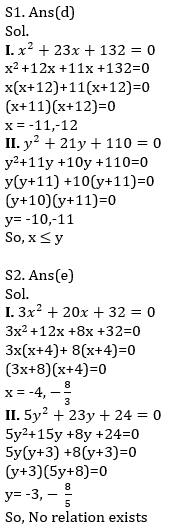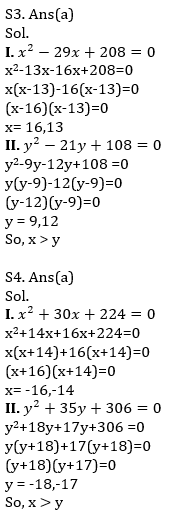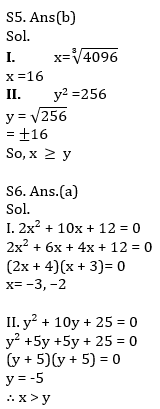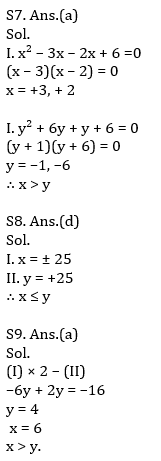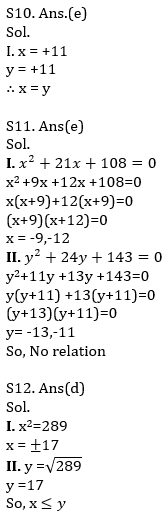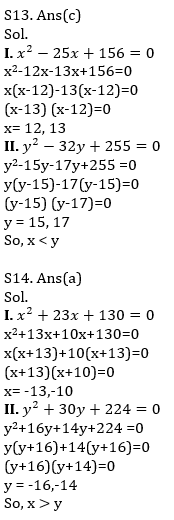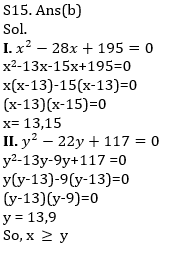Latest Banking jobs   »   Quantitative Aptitude Quiz For IBPS Clerk...

# Quantitative Aptitude Quiz For IBPS Clerk Prelims 2021- 23rd July

Directions (1-5):- In each of the following questions, two equations (I) and (II) are given. Solve the equations and mark the correct option:
(a) if x>y
(b) if x≥y
(c) if x<y
(d) if x ≤y
(e) if x = y or no relation can be established between x and y.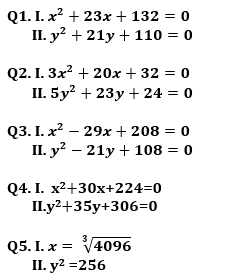Directions (6-10): In each of these questions, two equations (I) and (II) are given. Solve the equations and mark the correct option: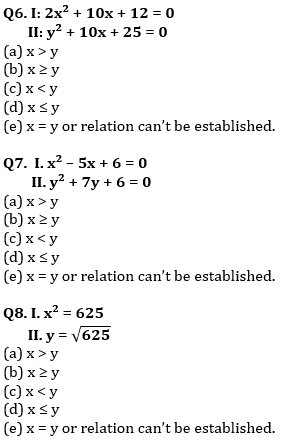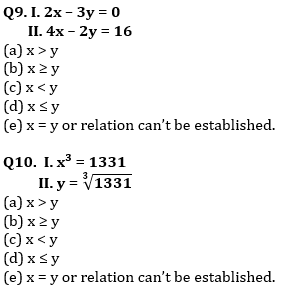Directions (11-15): In each of the following questions, two equations (I) and (II) are given. Solve the equations and mark the correct option:
(a) if x>y
(b) if x≥y
(c) if x<y
(d) if x ≤y
(e) if x = y or no relation can be established between x and y.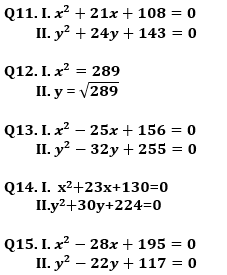Solutions